## //----------------------------// ArtMatic 1 in 3 out components //----------------------------//

Set Constant
Set Vector (Ax,Bx,Cx)
3D derivative dx,dy,dz
Unpack
RGB gamma
RGB half plane
RGB Neon line
Multi Perlin noise
Random color
Hue color
Color Sines
Linear motion
Circular motion
Random motion
Timeloop Interpolator
13 Compiled tree

## Introduction

1->3 Components generally serve one of three functions: to convert a scalar (single value) stream to RGB color, to generate three independent values, or to unpack an RGB stream.

##13 Set Constant

parameters :
A : X or Red Value (0. : 1.)
B : Y or Green Value (0. : 1.)
C : Z or Blue Value (0. : 1.)

discussion :
Create three independent constant values whose values are determined by parameters A, B, C and the chosen Algorithm. The input value is ignored. The output can be thought of as either three independent values or as a single RGB color.

algorithms :

##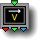13 Set Vector (Ax,Bx,Cx)

parameters :
A : A (-12. : 12.)
B : B (-12. : 12.)
C : C (-12. : 12.)

discussion :
Scale the input value using parameters A, B and C. Output is A*x, B*x, C*x.

##13 Add (x+A,x+B,x+C)

parameters :
A : A (-50. : 50.)
B : B (-50. : 50.)
C : C (-50. : 50.)

discussion :
This component is sometimes referred to as Add Vector, the constant vector added being (A,B,C). The three outputs are x+A, x+B and x+C. Add Vector can be useful for adding offsets to the same axis to move several objects in the same axis.

##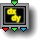13 3D Derivative dx,dy,dz

parameters :
A : Amplitude (-8. : 8.)
B : Normalize % (0. : 1.)

discussion :
Returns the partial derivatives dX, dY and dZ of the input function (which shall be a 3D function). When the algorithm is set to "VY Object/Terrain Normale" the normal is given directly from ArtMatic Voyager rendering in the shading phase and the input is ignored. This component is useful to implement custom shaders and textures for 3D DF objects and terrains. 'Amplitude' is usually kept at 1 but you can set it at -1 to reverse the normal. 'Normalize' can be used to force the normal to be of 1 magnitude.

algorithms :
• Compute Normal:
Partial derivatives of 3D input function. The derivatives are taken by evaluating the tree above several times using finite differences. The Derivative component has to "see" the function above which means it cannot be used within a CT to compute the derivative of a function depending on values computed outside. Practically if you create a CT for a function and needs its derivative, put the derivative component outside the CT and connect the derivative to the CT output.

• VY Object/Terrain Normal
The Normale is provided by Voyager in the shading phase. It is unavailable for the object geometry definition or terrain elevation, because they are providing the normal once rendered.

parameters :
No parameters.

##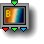13 Main Gradient

parameters :
A : Gradient Scale X (0. : 8.)
B : Gradient Offset (-2. : 2.)

discussion :
Main Gradient maps input value to a color of the main gradient (the current gradient). When parameters A 'Scale' and B 'Offset' are 1 and 0 respectively, 0 maps to the leftmost color of the gradient and 8 maps to the rightmost color.

algorithms :
• Linear:
Input values maps to gradient colors using a linear ramp. 'Scale' sets the ramp slope.
• Linear mirror:
Like previous mode but the gradient is mirrored for negative x.
• Logarithmic:
Logarithmic uses 1+log(x+1/e) so that 0 maps to zero.
• Logarithmic mirror:
Logarithmic Mirror uses ABS(x) to feed the logarithm. This mode corresponds roughly to the Logarithmic clut mode for scalar 1D output system.
• Dual Logarithmic:
This mode corresponds to the Balanced log clut mode for scalar 1D output system. It reverts the hues of the gradient for negative x;
• Cyclic sin
Cyclic sin uses a sine wave to loop the input. Thus the gradient progresses back and forth indefinitely. 'Scale X' controls the period and 'Offset' the phase. This mode corresponds roughly to the Cyclic clut mode for scalar 1D output system. In 8.08 the entire gradient is looped whatever the period.
• Cyclic mod(ulo)
Cyclic modulo uses a saw tooth wave to loop the input before mapping into the gradient colors. As a result the gradient progresses forwards only while looping so to achieve continuity you'll need that the first color matches the last color. 'Scale X' controls the period and 'Offset' the phase. In 8.08 phases are adjusted so that first instance overlaps the non looping mode.

##13 Indexed Gradient

parameters :
A : Scale X (0. : 8.)
B : Offset (-2. : 2.)
C : Index (0 : 48)

discussion :
This component is similar to
13 Main Gradient but uses parameter C (Index) to choose the gradient that will be taken from the built-in Gradient list.
Starting engine 8.08 Indexed Gradient has the same algotithm options than 13 Main Gradient.

algorithms :
• Linear:
Input values maps to gradient colors using a linear ramp. 'Scale' sets the ramp slope.
• Linear mirror:
Like previous mode but the gradient is mirrored for negative x.
• Logarithmic:
Logarithmic uses 1+log(x+1/e) so that 0 maps to zero.
• Logarithmic mirror:
Logarithmic Mirror uses ABS(x) to feed the logarithm. This mode corresponds roughly to the Logarithmic clut mode for scalar 1D output system.
• Dual Logarithmic:
This mode corresponds to the Balanced log clut mode for scalar 1D output system. It reverts the hues of the gradient for negative x;
• Cyclic sin
Cyclic sin uses a sine wave to loop the input. Thus the gradient progresses back and forth indefinitely. 'Scale X' controls the period and 'Offset' the phase. This mode corresponds roughly to the Cyclic clut mode for scalar 1D output system. In 8.08 the entire gradient is looped whatever the period.
• Cyclic mod(ulo)
Cyclic modulo uses a saw tooth wave to loop the input before mapping into the gradient colors. As a result the gradient progresses forwards only while looping so to achieve continuity you'll need that the first color matches the last color. 'Scale X' controls the period and 'Offset' the phase. In 8.08 phases are adjusted so that first instance overlaps the non looping mode.

##13 Shaded plain color #

parameters :
A : Red (0. : 1.)
B : Green (0. : 1.)
C : Blue (0. : 1.)
D : Contrast % (0. : 500.)

discussion :
This component maps input value to a shade of the color defined by parameters Red,Green,Blue. or a shade of its complementary color. The parameter D 'Contrast' scales the input prior the luminance shading of the color. With Ramp the shading uses D*|x| while with Contrast the shading uses clamp(mix(1,x,D),0,1) meaning a value of 0 for D will means no shading takes place.

algorithms :
• Dual color (legacy):
Negative inputs maps to the complementary color while regions closing to zero are faded to black. The 'Contrast' parameter scales the shading symmetrically around zero.
• Single color contrast:
• Dual color contrast:
Similar to Dual color but the 'Contrast' parameter scales the shading downward. At zero no shading takes place and the output is the constant color given by parameters (A,B,C).
• Dual Aux color A contrast:
Uses Aux Color A for negative x instead of complementary color.
• Dual Aux color B contrast:
Uses Aux Color B for negative x instead of complementary color.
• Dual color ramp:
Uses Aux Color B for negative x instead of complementary color and uses black to shade region near zero x. brightness shading uses uses D*|x|
• Triple color ramp
Uses Aux Color B for negative x instead of complementary color and uses Aux Color A instead of black to shade region near zero x. Practically it gives you a 3 color ramp as a procedural gradient. brightness shading uses uses D*|x|

##13 RGB gamma

parameters :
A : Gamma red (0.12 : 8.)
B : Gamma green (0.12 : 8.)
C : Gamma blue (0.12 : 8.)

discussion :
Map a value to RGB with independent control of the R, G and B channels response curve. Gamma is an efficient way to “tint” monochrome image or to give linear grey shades a colored neon light look.

##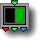13 RGB half plane

parameters :
A : Amplitude (-8. : 8.)
B : Offset (-16. : 16.)
C : Color Cycle (0. : 1.)
D : Color Saturation (0. : 1.)

discussion :
This component can be seen as either a simple color shader or a mathematical primitive. When used as a color shader, it generates a ‘half-plane’ of the color defined by Parameter C. When the offset is 0, the color is generated wherever the input value times Parameter A is negative; everywhere else is black. There is a smooth transition from black to the defined color. With non-zero settings of parameter A that are close to 0, the transitional area increases in size. This transition area may only be visible when zoomed far in or with amplitude values very close to 0. When 'Amplitude' is 0, the output is the color defined by Parameter C. When A < 0, the color appears on the right. When A > 0, the color appears on the left. Advanced users can use this component to implement complex systems that behave differently for negative and positive input values.

Example 1: A Half-plane connected directly to the Global X generates a half-plane of the color defined by Color Cycle:Example 2: When connected to a surface, the result is a virtually two-tone pattern with no intermediate shades except where the input value is very close to 0.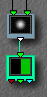##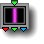13 RGB Neon line

parameters :
A : Red (0. : 1.)
B : Green (0. : 1.)
C : Blue (0. : 1.)
D : Spread % (0. : 1.)

discussion :
This is a simple color shader that generates a vertical horizontal line if fed by the global X input or a horizontal line if fed by the global Y input. The component can also be thought of as a complex filter that outputs its maximum value (as an RGB color) where the absolute value of its input is 0 and generates increasingly smaller values as the absolute value of its input increases. When attached to the output of surface, the result is a monochrome image made up of shades of the color defined by parameters A, B and C.

##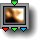13 Multi Perlin noise

parameters :
A : Amplitude (-8. : 8.)
B : Octaves (1. : 128.)
C : Frequency (0. : 32.)
D : Roughness (0.12 : 0.75)

discussion :
13 Multi Perlin Noise returns a 3D Vector of
Multi Perlin noise with different phases for each components. It can be seen as a triple 11 Multi Perlin Noise function. As with the 11 version the "Octaves" parameter controls the amount of higher frequencies additions and the 'Roughness' parameter the fractal dimension of the resulting noise. Values are balanced and can become negatives so when using it as a random color generator you will need to scale and offset the values.

##13 Random color

parameters :
A : Color Variance (-2. : 2.)
B : Saturation (0. : 1.)
C : Luminance (0. : 1.)

discussion :
Color shader that performs a random mapping of the input value to an RGB color whose saturation and luminance are determined by parameters B and C. The color range is determined by parameter A. The output is smoothed so that there are smooth transitions from color to color when the input is continuous.

##13 Hue color

parameters :
A : Hue Variance (0. : 8.)
B : Saturation (0. : 1.)
C : Luminance (0. : 1.)

discussion :
Color shader that maps input to a color defined by the HLS values defined by parameters A, B and C. The saturation and luminance of the output color are determined by parameters B and C. Parameter A, Hue Variance, is multiplied with the input value before the being mapped to a hue value. As a result, when Hue Variance is small, this component is not very sensitive to changes in the input value. When Hue Variance is large, the component is very sensitive to input value changes. The output is periodic.

##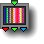13 Color Sines

parameters :
A : Red freq (-12.57 : 12.57)
B : Green freq (-12.57 : 12.57)
C : Blue freq (-12.57 : 12.57)

discussion :
Color shader that maps the input value to an RGB color by applying independent sine functions to the R, G and B outputs. This can be useful for creating sounds.

##13 Linear motion

parameters :
A : Direction x (-1. : 1.)
B : Direction y (-1. : 1.)
C : Direction z (-1. : 1.)
D : Speed (-50. : 50.)

discussion :
"Linear motion" provides a 3D linear motion akin to the 12
Linear motion tile when input is connected to time (w) or z input in 2D systems. The direction is given by the "Direction" vector parameter that will be normalised internally. Use (0 ,-1,0) to move upward for example. The 'Speed' parameter scales the input time. When negative, motion will proceed backward. The motion is at constant speed if the input is linear. The resulting position has to be added to the target coordinates to actually move it, usually with a packed 3D vector add like Packed RGB Add or better Packed Vector Maths when the target is a 3D object.

##13 Circular motion

parameters :
A : Radius (0. : 100.)
B : Angle oX (-180. : 180.)
C : Angle oZ (-180. : 180.)
D : Speed % (-50. : 50.)

discussion :
When the input is connected to time (w), the result is a circular motion in 3D. With angles oX and oZ at zero the circle is on the XZ plane. You may rotate the circle plane with the 'Angle oX' and 'Angle oZ' parameters (in degree). The 'Radius' sets the radius of the circle.The 'Speed' parameters scales the input time. When negative, motion will proceed backward.
The motion position produced by Circular motion has to be added to the target coordinates usually with a
Packed Vector Maths tile when the target is a 3D object.
The tile has the options to interpret the radius in various units.
Examples : Libraries/Components demo/Motion3DMetaballs.artm

##13 Random motion

parameters :
A : Radius (0. : 100.)
B : Speed % (-50. : 50.)

discussion :
This component creates continuous random 3D motion when the input is connected to time (w). 'Radius' sets the radius of the motion and 'Speed' parameter scales the input time. When negative, motion will proceed backward. The random motion path is generated using a 1D vector valued (3D) perlin noise function.The motion position produced by Random motion has to be added to the target coordinates usually with a a
Packed Vector Maths tile when the target is a 3D object.
The tile has the options to interpret the radius in various units.
Examples : Libraries/Maths/Functions plots/RandomMotion3D.artm

##13 Timeloop Interpolator

parameters :
A : Period (0.12 : 32.)

discussion :
Timeloop Interpolator is a utility that makes creation of seamless loops easy.
It outputs 2 overlapping cycling ramps in first 2 outputs with a blending function in third output (z). The blending function shall be used to fade whatever the 2 cycling ramps controls so that looping is continuous. Usually you will use a 31 interpolation tile to blend the 2 functions with z controlling the interpolation like
31 z Blend and the Timeloop tile will be connected to time. If the function to loop is RGBA use Packed z Blend and pass z to the alpha input. The looping take place over the given period so set the animation time to an integer multiple of the period to have 1 or several loop instances.
With Timeloop Interpolator you can create continuous periodic signals with anything as well as seamless motion loops.
Examples : Libraries/Animated Loops/FiberWaves Loop 16.artm Libraries/Animated Loops/Gamma Clouds PanX.artm Libraries/Maths/Periodic fractal signal.artm

parameters :
A : Scale 0:1
B : Iterations